Get instant live expert help with Excel or Google Sheets“My Excelchat expert helped me in less than 20 minutes, saving me what would have been 5 hours of work!”

#### Post your problem and you’ll get expert help in seconds.

Your message must be at least 40 characters
Our professional experts are available now. Your privacy is guaranteed.

# Here is How You Can Perform NPV Break Even Analysis in Excel

While working with excel, a time comes when we are required to get the break-even analysis for the firm or organization we are working for. To do this, we need to use the goal seek method to solve it. Here is a how you can perform the NPV break even analysis in excel using the goal seek method.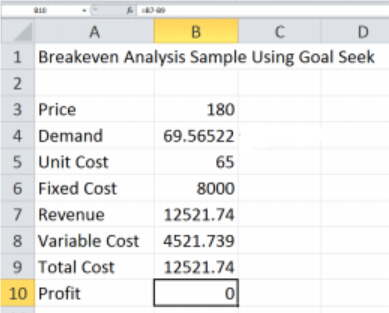Figure 1: How to find break even analysis in excel

## Tips on how to get the NPV break even analysis in excel

To understand how to perform the analysis, follow the below example which highlights the procedure necessary for a successful NPV break even analysis.

### Step 1: Prepare the table with all the variable.

In our table, we shall have the following variables; price, demand, unit cost, fixed cost, revenue, variable cost, total cost and profit as shown in the table below;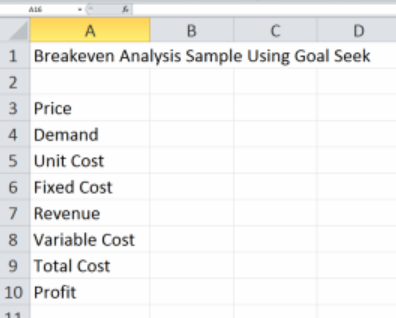Figure 2: Indicate all the variable required

### Step 2: Enter the formulas into the table

We shall have the following formulas put in various cells in the table:

• Variable cost- (demand x unit cost) = B4*B5. This should be in cell B8. Ones entered, we have to click on the checkmark icon.
• Total cost- (fixed cost + variable cost)= B6+B8). This is put in cell B9, then click the checkmark icon.
• Revenue –(demand x price)= B4*B3. This should be in cell B7, and click the checkmark icon.
• Profit- (Revenue –total cost)= B7-B9. Enter this in cell B10 and click the checkmark icon.
• These are as shown in the figure below;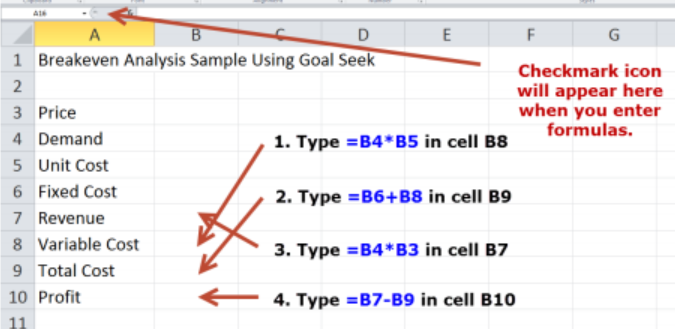Figure 3: Enter the various formula

The result of the above process should be a table that looks like the one below;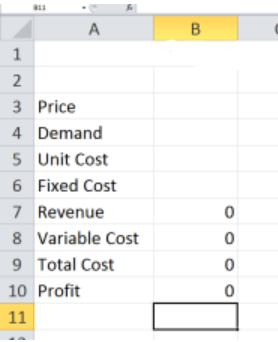Figure 4: How the table looks after you’ve entered the formula

To actually see how this formula works, we shall look at a firm that produces construction materials. The fixed cost is \$8, 000, unit cost is \$65, price is \$180 each. Note that when using the Goal seek process, demand is set to be 1. We need to enter this data in our table. After filling the table, it will look like the one below;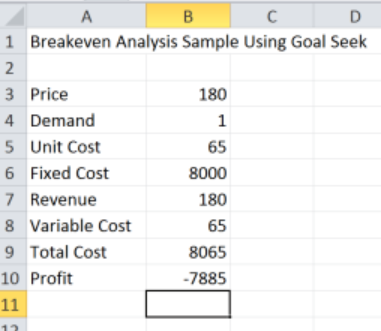Figure 5: How the table looks like with the data

Then get the what-if analysis. The next step that we need to do is select the profit cell, which in our case is B10, head to data and then click the What-If analysis. Select the Goal Seek from the dropdown menu in What-If analysis. We will get a popup window like the one below;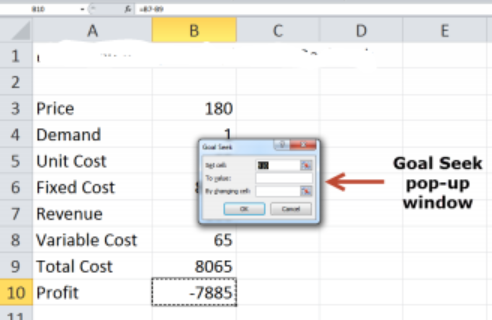Figure 6: How to get the Goal Seek

After that, type 0 next to the To Value and then enter \$B\$4 next to By changing Cell. Click ok twice afterwards. Ones you do that, you will get the approximate number of units of demand to break even. In our case, the number is approximately 70 units.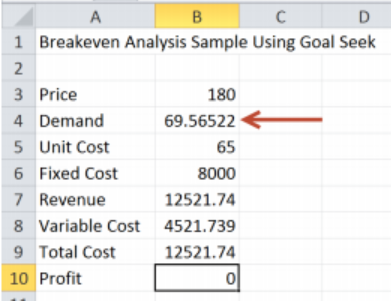Figure 7: Final result of the process

## Instant Connection to an Expert through our Excelchat Service

Most of the time, the problem you will need to solve will be more complex than a simple application of a formula or function. If you want to save hours of research and frustration, try our live Excelchat service! Our Excel Experts are available 24/7 to answer any Excel question you may have. We guarantee a connection within 30 seconds and a customized solution within 20 minutes.

Solution examplesI have a figure which is the sum of 9 months of payments. Each payment decreases by the same amount (X) so the first payment is Y, second payment is Y-X, third is Y-(Xx2), forth is Y-(Xx3) and so on. I am trying to calculate what each of the 9 payments should be.
Solved by A. W. in 60 mins